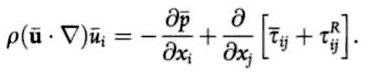## Monday, January 30, 2012

### The connection between Formula 1 and Dark Energy

Perhaps to the surprise of many aerodynamicists, one of the concepts deeply familiar to them, may actually explain the existence of dark energy.

This ominous form of energy corresponds to an extra source term in the cosmological version of the Einstein field equations, and is commonly held to be responsible for the accelerating expansion of the universe.

The connection with aerodynamics lies with a concept used to represent turbulent flow, called Reynolds Averaging. Despite the supercomputer power available in Formula 1, it remains impractical to solve the exact Navier-Stokes equations for a turbulent flow. Instead, the velocity and pressure fields are split into the sum of a mean field and a fluctuating component, and the Reynolds-Averaged Navier-Stokes equations are solved to calculate the mean fields.

However, the process of taking the average of the Navier-Stokes equations generates an extra term, which is interpreted as an extra source of stress. One thinks of the mean field as having to do work against the extra stresses created by turbulent eddies, as well as the viscous stresses already inherent in the fluid.

Thus, whilst the Navier-Stokes equations for a steady flow are as follows:The Reynolds-Averaged equations pick up an extra term on the right-hand side:Now, as cosmologist George Ellis and his colleagues explain, whilst the exact Einstein equations hold at the local scale:Once one averages the matter-energy and geometry over galactic scales, the Einstein equations pick up an extra term:Sound familiar? As Ellis et al comment, this "is the effective matter source term representing the effect of averaging out smaller scale structures."

Whether this type of effect can explain the quantitative cosmological data remains to be seen, but perhaps aerodynamicists have something to offer the cosmological community here.